Chemistry Exercises-The compound iron(lll) fluoride is a strong electrolyte. Write the transformation that occurs when solid iron(lll) fluoride dissolves in water
 (Referencesl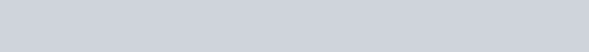Use the References to access important values if needed for this question.The compound iron(lll) fluoride is a strong electrolyte. Write the transformation that occurs when solid iron(lll) fluoride dissolves in waterwater.Next)
 Use the References to access important values if needed for this question.The compound zinc chloride is a strong electrolyte. Write the transformation that occurs when solid zinc chloride dissolves in water.Be sure to specify states such as (aq) or (s).2 more group attempts remaining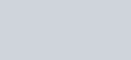Previous Next

Use the References to access important values if

The compound nickel(ll) nitrate is a strong electrolyte. Write the transformation water.

needed for this that occursquestion.

when solid nickel(ll) nitrate dissolves inPrevious

Be sure to specify states such as (aq) or (s).Retry Entire Group

2 more group attempts remaining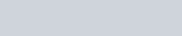the important

Use References to access values if needed for this question.

Classify each of the following as a strong acid or a weak acid and indicate how each should be written in aqueous solution.

In solution this acid should be written as:

Classify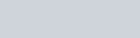1. hydrobromichydrochloric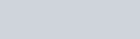2.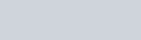3. phosphoric

 Retry Entire Group1 more group attempt remainingPrevious

Next)Use the References to access

Classify each of the following as a Strong acid (sa) or a Weak acid

Classify

important values if

(wa) and

In solution

remaining

this

needed for question.

indicate how each should be written in aqueous

this acid should be written as: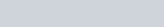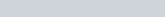1. hydrofluoric acid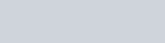2. hydrocyanic acid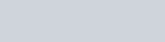3. nitric acid

 Retry Entire Group1 more group attemptsignmen

ova

Use the References to access important values if needed for this question.

Identify each of the following as a strong electrolyte, a weak electrolyte or a nonelectrolyte, based on its behavior when dissolvH20 2+

ca (aq) + 20H (aq)

CH30H(t) CH30H(aq)

HN02(aq) + H20(t) H30+(aq) + N02- (aq) HI(g) + H20(E) H30+ (aq) + r (aq)

 Retry Entire Group3 more group attempts remainingUse the References to access important values if needed for this question.

Write formulas for the major species in the water solution of each compound.

If the compound does not ionize, write as oiven. If more than one species, separate by commas. Do not write coeffients with ions.

 Compound Type Major Species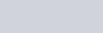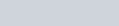strong electrolyte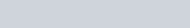nonelectrolyte 11CIO weak electrolyte HBr strong electrolyte
 Retry Entire Group3 more group attempts remainingtoclassifymore access important valuesifeach of the compounds as soluble or not soluble:group attempts remaining Use the ReferencesUsing the solubility rules on the information page, manganese(ll) chloride v calcium sulfide lead phosphate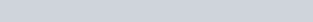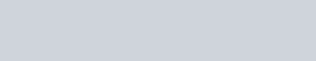3 Submit Answerteaching and lea X carbonic acid strong or weak-G X I Owl – Google Docs ssignment/takeCovalentActivity.do?locator=assignment-take [Review Tonics]Use the References to access important values if needed for this question.Write the net ionic equation for the precipitation of lead(ll) sulfate from aqueous solution:3 more group attempts remaining In progressSubmit Retry Entire Group 3 more group attempts remaining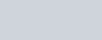Pause Use the References to access important values ifFor the following reaction, 4.18 grams of oxygen gas are mixed with excess carbon dioxide .carbon monoxide(g) + oxygen(g) carbon dioxide(g)What is the ideal yield of carbon dioxide?What is the percent yield for this reaction?AnswerRetry Entire Group more group attempt remaining needed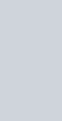formonoxide this question.. The reaction yields 8.34 grams of carbonpreviOUS Next) Submit1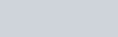[Review Topics] [References]Use the References to access important values if needed for this question.For the following reaction, 5.54 grams of potassium hydrogen sulfate are mixed with excess potassium hydroxide. The reaction yields5.35 grams of potassium sulfate.potassium hydrogen sulfate (aq) + potassium hydroxide (aq) —»potassium sulfate (aq) + water (l)What is the ideal yield of potassium sulfate?What is the percent yield for this reaction?1 more group attempt remaining